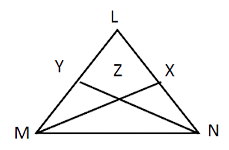# Triangles Practice Problems: Level 01

Q1. In ∆XYZ, M and N are points on XZ and YZ respectively such that MN is the perpendicular bisector of YZ. If XM = XY and ∠XYZ = 90, what is ∠XZY?
60°
30°
55°
45°
65°
Q2. In ∆LMN, medians MX and NY are perpendicular to each other and intersect at Z. If MX = 8 cm, NY = 12 cm, what is the area of ∆LMN?A. 24 cm2
B. 64 cm2
C. 48 cm2
D. 32 cm2
E. 56 cm2
3. The sum of all the three sides of an equilateral triangle is 72 &redic;3 cm. Find the height of this triangle.
A. 124 cm
B. 48 cm
C. 36 cm
D. 144 cm
E. 224 cm
4. In triangle PQR, S and T are midpoints of sides PR and PQ respectively. The medians QS and RT intersect at U. Then area PQU: area PQR is
A. 1 : 3
B. 1 : 4.
C. 1 : 5
D. 2 : 5
E. 3 : 4
5. If there is an equilateral triangle having area A and there is a square having an area B and they have the same perimeter, then
A. X>Y
B. X<Y
C. X = Y
D. XY
E. X ≤ Y
Q.6. The sum of three sides of an isosceles triangle equals 14m; the ratio of lateral side to the base is 5 is to 4. Find the area of the triangle in square meters.
A. 4&redic;21
B. 3&redic;3
C. 4&redic;3
D. 5&redic;3
E. 2&redic;21
Q.7. A flag pole, which is 36.2 ft high, casts a shadow of 5 ft at 11 a.m. The shadow cast by a tower at the same time is 35 ft long. The height of the tower is
A. 153.4 ft
B. 203.4 ft
C. 200 ft
D. 186.3 ft
E. 253.4 ft
Q8. PQR is a triangle. If S is a point in the plane of the triangle such that the perpendicular distance from S to the three sides of the triangle are all equal, then there exist(s)
A. Just one such point S
B. Three such points S
C. Two such points S
D. Four such points S
E. None of the above
Q9. If the area of triangle ABC is 1/4 (a2+b2) where a and b are the lengths of two sides, find the angles of the triangle.
A. 30°, 60°, 90°
B. 60°, 60°, 60°
C. 90° , 45°, 45°
D. 45° , 60°, 45°
E. None of these
Q10. The length of the three sides of a triangle is in the ratio of 5:12:13. The difference between largest side of this triangle and the smallest side of this triangle is 1.6 centimeters. Find the area of the triangle?
A. 2.4 cm2
B. 30 cm2
C. 1.2 cm2
D. 1.8 cm2
E. 1.5 cm2